# AI theory - maybe useful for bots in games?

2»

• Posts: 6,125Volunteer Moderator
That's one of the great things about tools like Unity, it makes it easier to rapidly test ideas.
That's really my favourite area of development: blue sky research. That's where you just try things out to see if you can make them work. I don't care about making a finished product, I want to prove ideas then let someone else worry about polish and support.I guess that's why I like being a teacher. My students come to me with questions like "how can I make a crab leave a trail in the sand as it walks around on an island?" Then I have to come up with an answer on the fly.
(The crab one was real)

• i took the time to detail two fighting methods; gun fighting, sword fighting, and made YouTube videos with diagrams that are animated to show what i mean.

how this relates to this thread is on December the 18 i detailed strategy for fighting, not the method. here i detail the method first for shooting which is simple, and then for sword fighting which is complex.

if you look at how i detail method 1 and 2 in sword fighting you see method 1 is complex or a example of the multiplication i detailed in this thread, a strength. while method 2 is additive.

this goes back to gun fighting, same mechanism but detailed more in depth in sword fighting.

so without further talk here are the two fighting methods i detailed. applicable to AI for problem solving.

guns:

"how to shoot a gun and hit a target in apex legends

first off there is three different types of movement while shooting; strafe left, strafe right, forward.

if you shoot starting with strafing left or right theres two different results, shooting from the good strafe side or shooting from the bad strafe side.

the good strafe side is the hand you use to write with and pulling the arm into your chest area.

then theres the optimal method, starting with moving forward and shooting, you then follow up each shot strafing with your good strafing side.

it works because of math, you have two directions to consider with strafing, and if it works like the writing hand then considering two directions with the writing hand is considerably easier than considering two directions with your non-writing hand.

and moving forward is only one direction.

moving forward is what sets up the strafing to be more likely to hit the target.

in the example its easiest to show this with the machine gun because of the bullets in the gun.

in game because of the confusion and surprise element you can imagine how hard it is to get this down correctly, when it doesnt you usually die.

a ninja is the legendary warrior who never forgets how to do this. i however do forget and die in the game regularly and easily. my memory and game sense is low.

"

swords:

"
how to sword fight

sword fighting using math.

person 1 cuts at person 2. person 2 has their sword and tries to block the strike using 1 of two methods.

method 1 is from the farther side of the body to the opponents sword, hit sword with sword.

method 2 is to use the sword from the closer side to the opponents sword.

postulating that method 1 takes skill where method 2 doesn't, if you have skill then try method 1, but you don't know maybe the opponent 1 is more skilled so you take a chance with method 1.

with method 1 you turn the torso to get power from the trunk of the body, but with method 2 you lean into the opponent 1 sword and thats it.

method 2 is also for less bright fighters, you don't need to be a genius to sword fight this way.

then the math comes is that as method 1 or 2 works, opponent 1 has two moves to consider, whereas opponent 1 can potentially only have 1 move to consider. the two moves opponent 1 has to consider are the to the side and toward the opponent, or contradicting lines, then opponent 2 seeing opponent 1 has 2 contradicting line directions to consider considers only one line to the opponent and that is to bring their sword onto the opponent.

now as opponent 2 goes to bring their sword down on opponent 1 there is the complicated method which is like baseball swings, or downward from the swing down over the head.

down from over the head is method 2, and baseball bat swinging is method 1 the harder method.

la poste di falcone the guard of the hawk
"

• i got around to making the initial flow chart for the math in this post:

see the attached file. open it at https://www.draw.io/

now i can expand that flow chart to include the larger math later on when im either not as busy as i am now or not as lazy as i am most of the time.

ill even attach a jpeg in case you dont want to open the flow chart;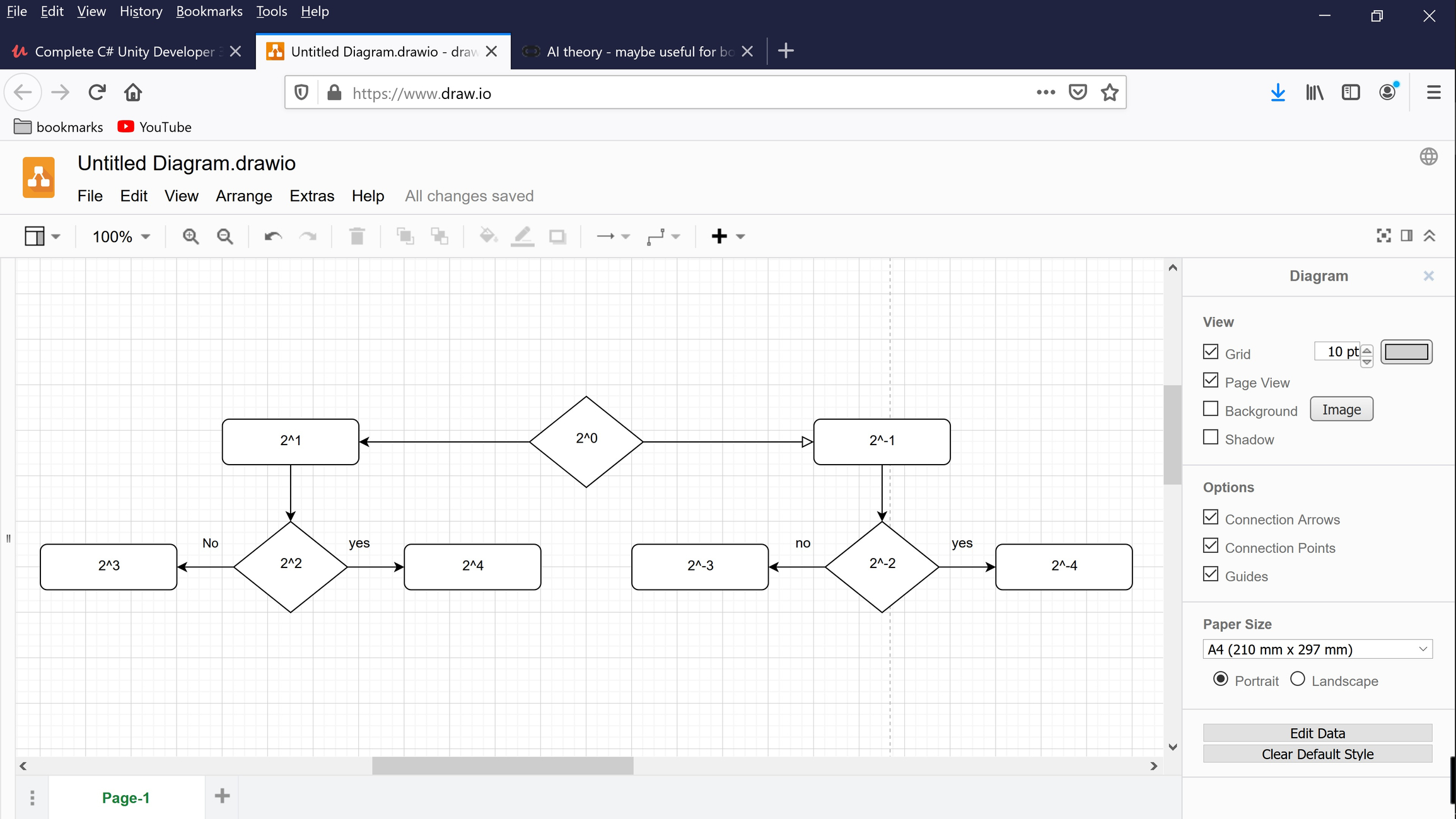• i got inspired and decided to make the bit i wrote about in this post;

here is the jpeg of the flow chart and attached is the flowchart you can open at https://www.draw.io/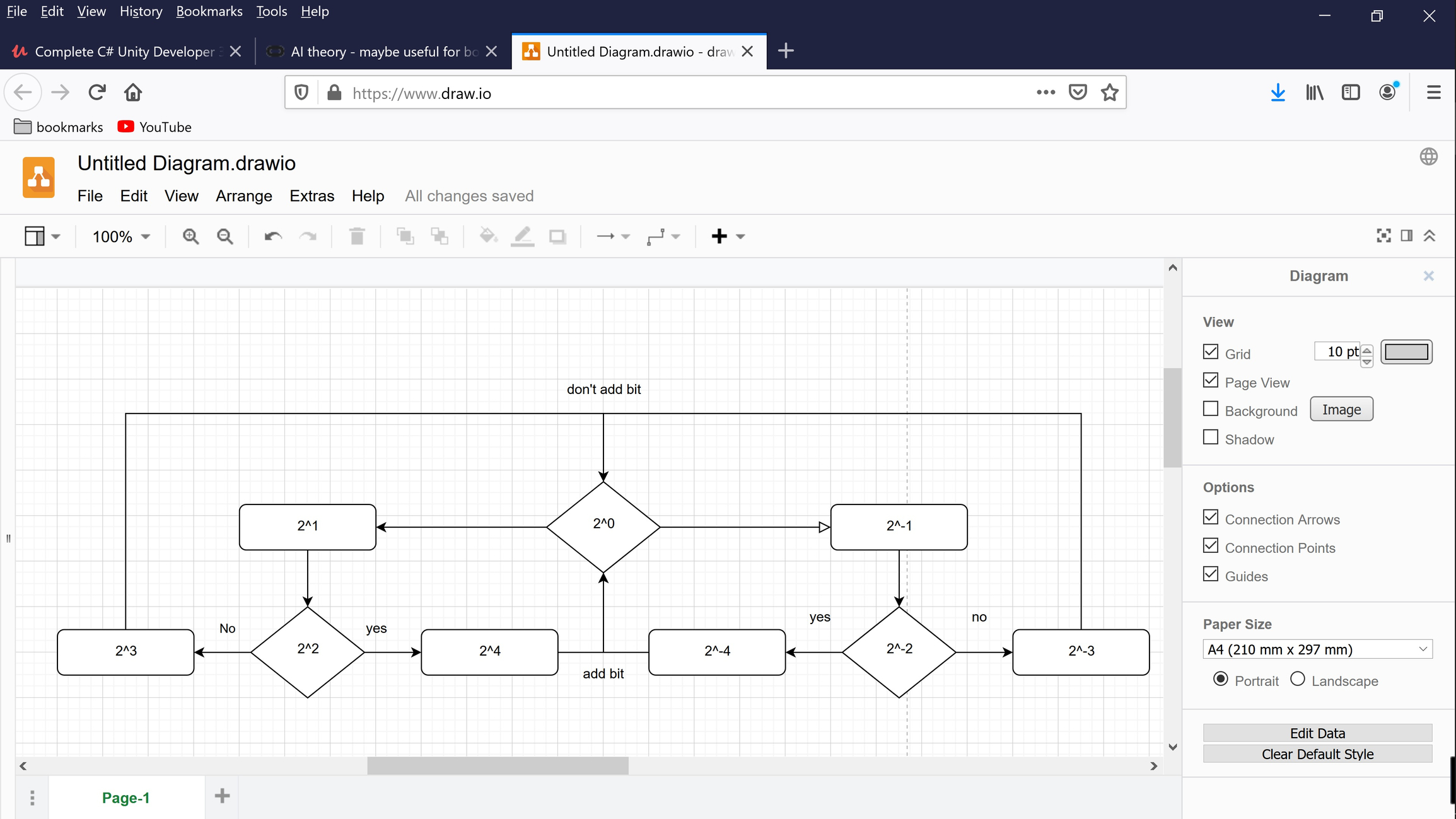• edited January 3
here is the work i  did to increment the binary, in the flow chart.
this only shows 2^0, 2^1, its a proof so i only did to 2^1.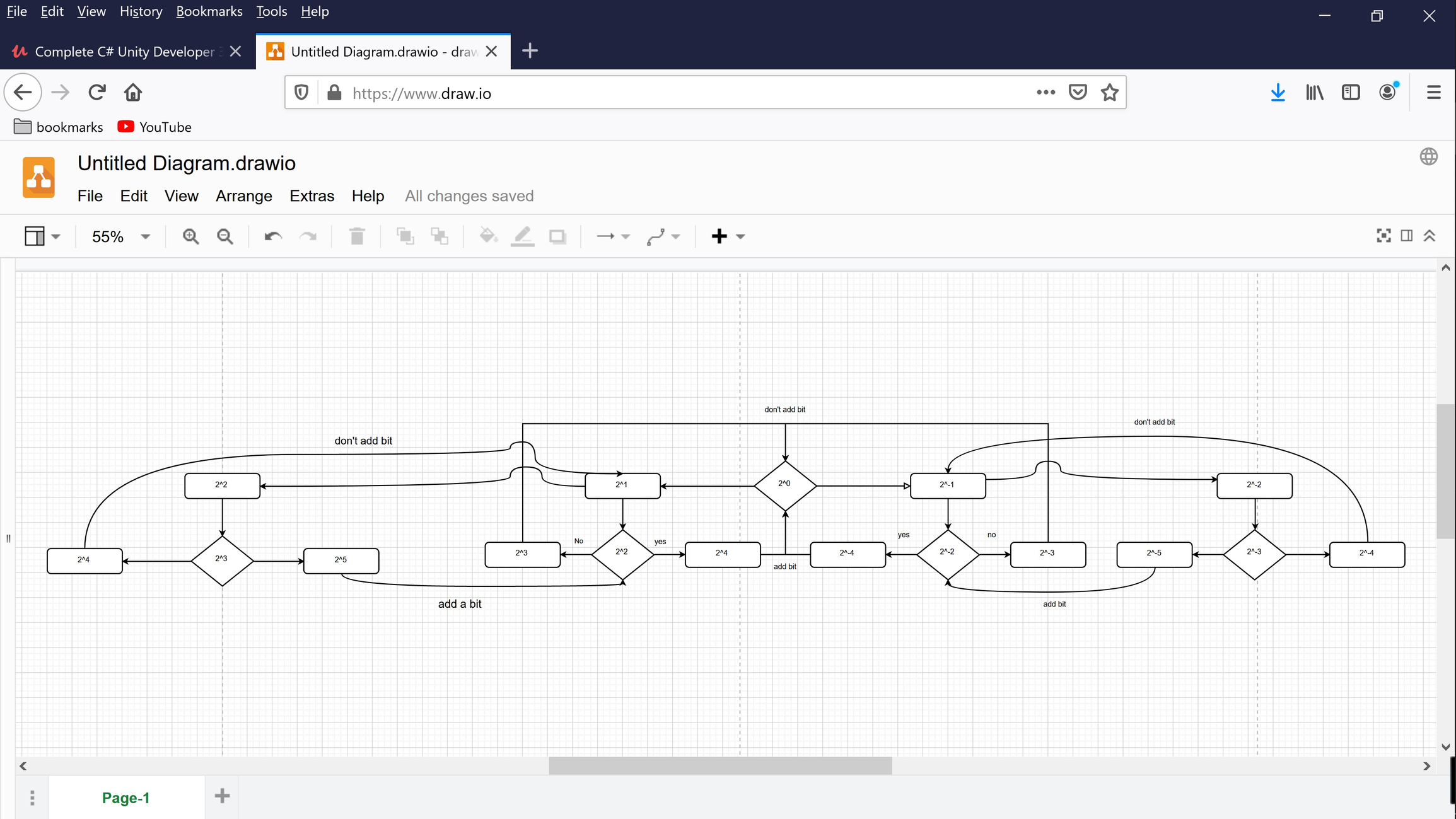this describes my posts in this thread up to but not including the box that's taped and then opened up. I'm quite lazy, quite slow, i might have to rest a long time to get enough interest to incorporate that into my flow chart then if i can get over how lazy and comfortable i am, i can work out the multiplicative and addition logic that's integral to fighting into the logic of the flowchart. i have very little hope of doing that considering how lazy i am. but we'll see.

• edited January 5

i made the math and diagram to solve the post about the taped box. see the attached picture and diagram you can open at draw.io

as you can see, the don't add bit becomes add bit. this solves for the taped box. 1 tape.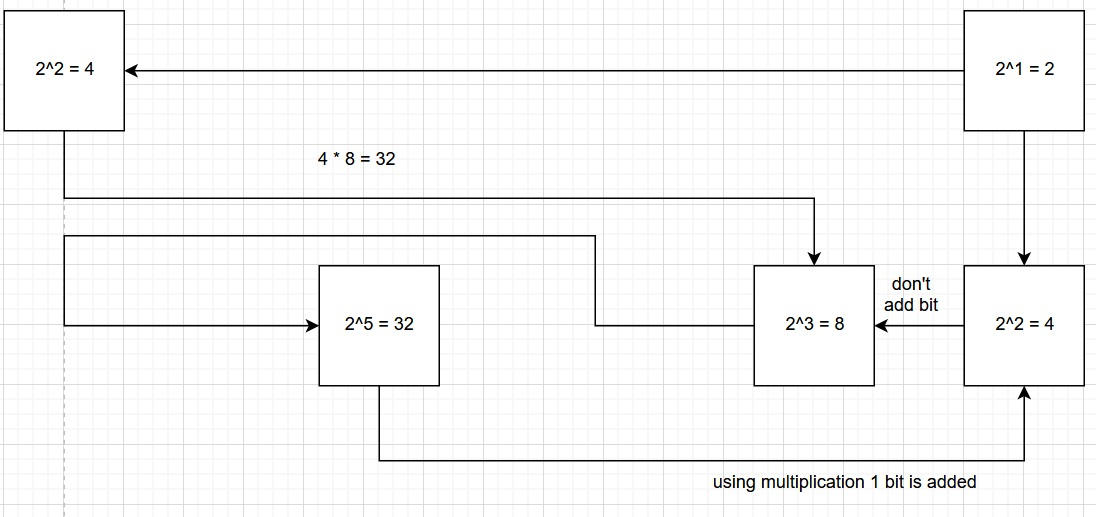add in the box lip under the tape that will be cut by the scissors and you increment 2^0 to 2^1. when you increment 2^0 then each bit in the diagram in incremented +1 also.

increment the bit values like this:

2^2 becomes 2^3 = 8
2^3 becomes 2^4 = 16
8*16 = 128
[
128 / 2 = 64
2^5 becomes (2^6 = 64)
]

then repeat this to increment the bit values;

2^3 becomes 2^4 = 16
2^4 becomes 2^5 = 32
16*32 = 512
[
512 / 4 = 128
2^6 becomes (2^7 = 128)
]

...

• Posts: 15,006Valuable Player
edited January 5
Yep, reminds me of a great essay I once read.

The great antiquity of Nothing is apparent from its being. Nothing, then, is not Something. And here I must object to a third error concerning it, which is, that it is in no place—which is an indirect way of depriving it of its existence; whereas, indeed, it possesses the greatest and noblest place upon this earth, viz., the human brain. As Nothing is not Something, so everything which is not Something is Nothing; and wherever Something is not, Nothing is—a very large allowance in its favour, as must appear to persons well skilled in human affairs. Nothing contains so much dignity as Nothing.  This is, indeed, the highest degree of Nothing, or (if I may be allowed the word) the Nothingest of all Nothings….

Are you a fan of the Myst games? Check out my Mod at http://www.mystrock.com/
• i drew the diagram to explain this post about multiplication corruption and addition goodness; https://forums.oculusvr.com/community/discussion/comment/719138/#Comment_719138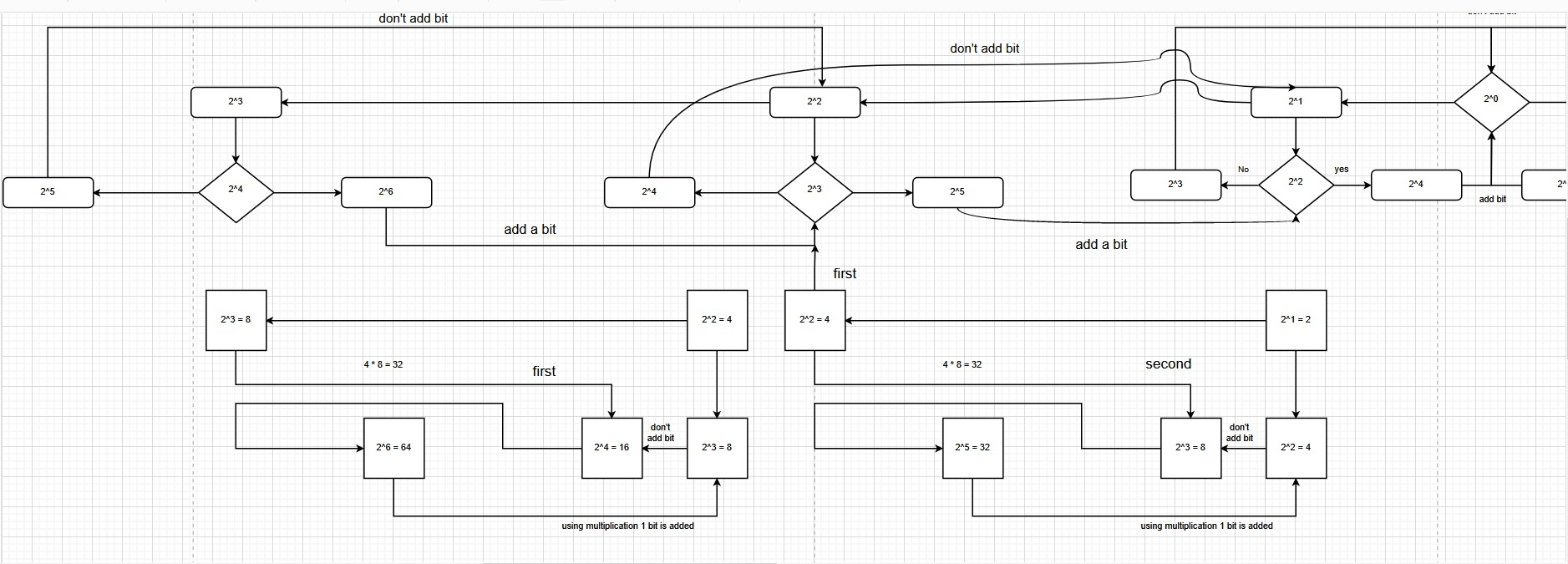multiplication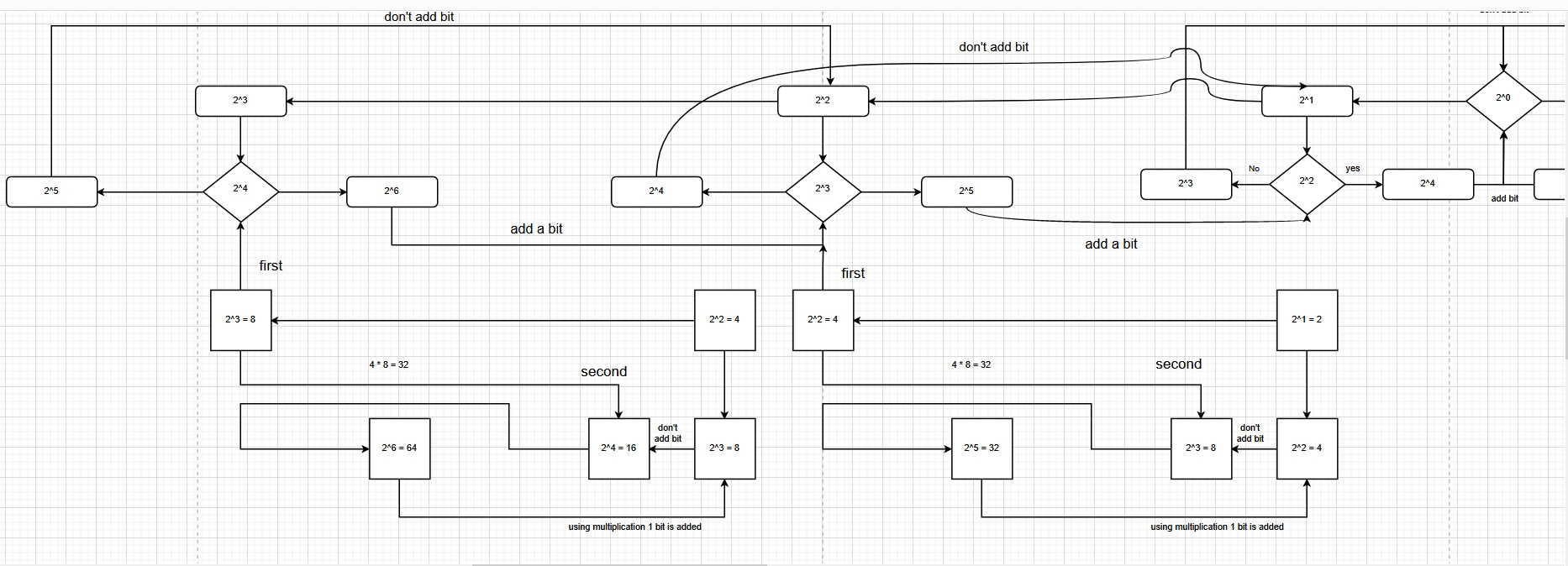see the attached zip file for the diagram file you can open at draw.io

- multiplication == true.

• in the final post before i start talking about fighting, here;

i showed this math;

([(->, <-), (->, <-)] + ->) = 0
([(->, <-), (->, <-)]  + (->, <-)) = 1
([(->, <-), (->, <-), (->, <-)] + (->, <-) + ->) = 0
([(->, <-), (->, <-), (->, <-)] + (->, <-) + (->, <-)) = 1
([(->, <-), (->, <-), (->, <-), (->, <-)] + (->, <-) + (->, <-) + ->) = 0
...
then i showed the conditions;

- multiplication == true.

using a geometric shape we have the uniform tube with a bloomed out tip.

the female goes from the bloomed out tip to the opposite end;

- multiplication == true.

the male goes from the non-bloomed out tip to the bloomed out tip;

the strafing logic is as multiplication  is not addition, it becomes addition, and this logic is true, male and female become 1;

([(->, <-), (->, <-)] + ->) = 0
([(->, <-), (->, <-)]  + (->, <-)) = 1

therefore as multiplication pushes addition (0, 1, 0), addition that is the male moves to counter this and eventually the bloom of the tube stops and the female goes to the male (0, 1), which works out like strafing in a fps game.

the female has to go both (0, 1, 0, 1) or wobbly strafing, while the male only has to go the one direction strafing (0, 1).

so to show this i would have to animate the diagram to bloom out to a tube and wide tip then shrink to its original shape.

i decided to draw it out so there's no confusion, here is the description;

- increment female first to 2^3
- increment female first to 2^4
- (male or) decrement female second to 2^4
- (male or) decrement female second to 2^3

in decrement female second to 2^3 there is no (increment female first to 2^3), there is only addition.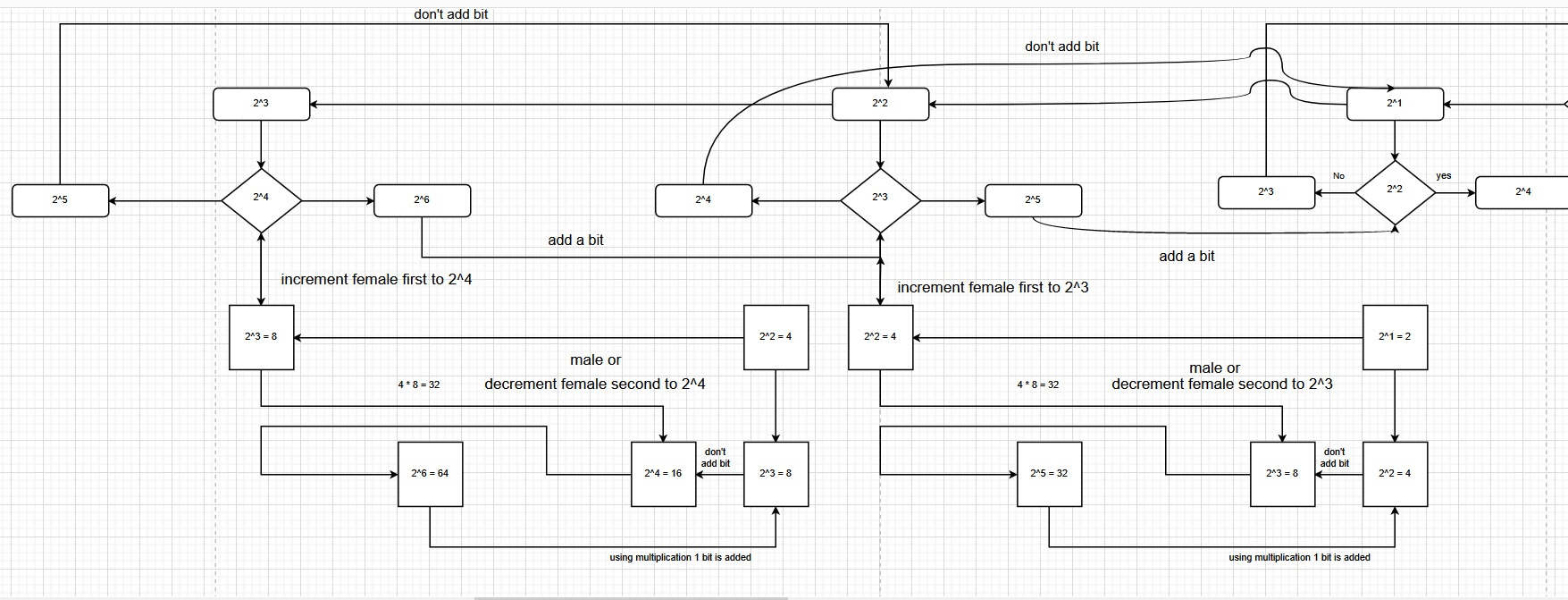i attached the file with the diagram and larger picture. from here i can incorporate the fighting methodology i describe here, or not. doing this work was hard. i think i will b lazy again. gud luck.

• i had to think how this post was made in the flow chart;

i decided there is a nest of while loops. the inner while loop increments a value, else the loop is exited, then that while loop stops being the inner while loop and the next closest while loop becomes the inner while loop, and this repeats until a winner is decided and all the while loops go away leaving only 1 while loop the winner.

to draw out the flow chart for this, since its strategy only, i would only need to work about 4 directions. in the flow chart i drew out the complete positive value chart, the negative values are basic and need to be updated to resemble the positive diagram.

then that takes care of the back and forth, the forward and back are the complex numbers that use the imaginary number line, starting from (i, -i). that would look line;

- [2i^0 = 2] = 2^1 = left
- [2i^1 = 2i] = forward
- [2i^2  = -2] = -2^1 = right
- [2i^3 = -2i] = reverse
- [2i^4 = 2] = 2^1 = left
- [2i^5= 2i] = forward
- [2i^6 = -2] = -2^1 = right

- [-2i^0 = -2]  = -2^1 = right
- [-2i^1 = - 2 i] = reverse
- [-2i^2 = 2] = 2^1 = left
- [-2i^3 = 2i] = forward
- [-2i^4 = -2] = -2^1 = right
- [-2i^5 =  -2i] = reverse
- [-2i^6 = 2] = 2^1 = left

the complex numbers only go into the first part of the diagram of the left and right side.

as the female grows out of proportion, the male uses the imaginary to create a partition in the left or right. this partition only going in to either 2 or -2 can't be contaminated to grow out of proportion.

then you have the pattern

inner loop
{
- [2i^0 = 2] = 2^1 = left ( out of proportion using real numbers)
- [2i^1 = 2i] = forward (in proportion using imaginary numbers)
- [2i^2  = -2] = -2^1 = right (in proportion using real numbers)
- [2i^3 = -2i] = reverse (get out of danger)
- [2i^4 = 2] = 2^1 = left (loop left)
- [2i^5= 2i] = forward (loop forward)
- [2i^6 = -2] = -2^1 = right (loop right)
}

if the inner loop is replaced, the companion to reverse is forward, repeat the same strategy as the previous inner loop.

and that would be the strategy.

i decided not to draw this out on the diagram because 1.) I'm confused how to do so, 2.) i am lazy and don't want to figure out how to do it correctly. so there u go.

accordion fake

• i drew it out, c the included folder for the diagram you can open at draw.io, and i took a snapshot of it i will upload here for you to gander at in case you don't want to open the file at draw.io;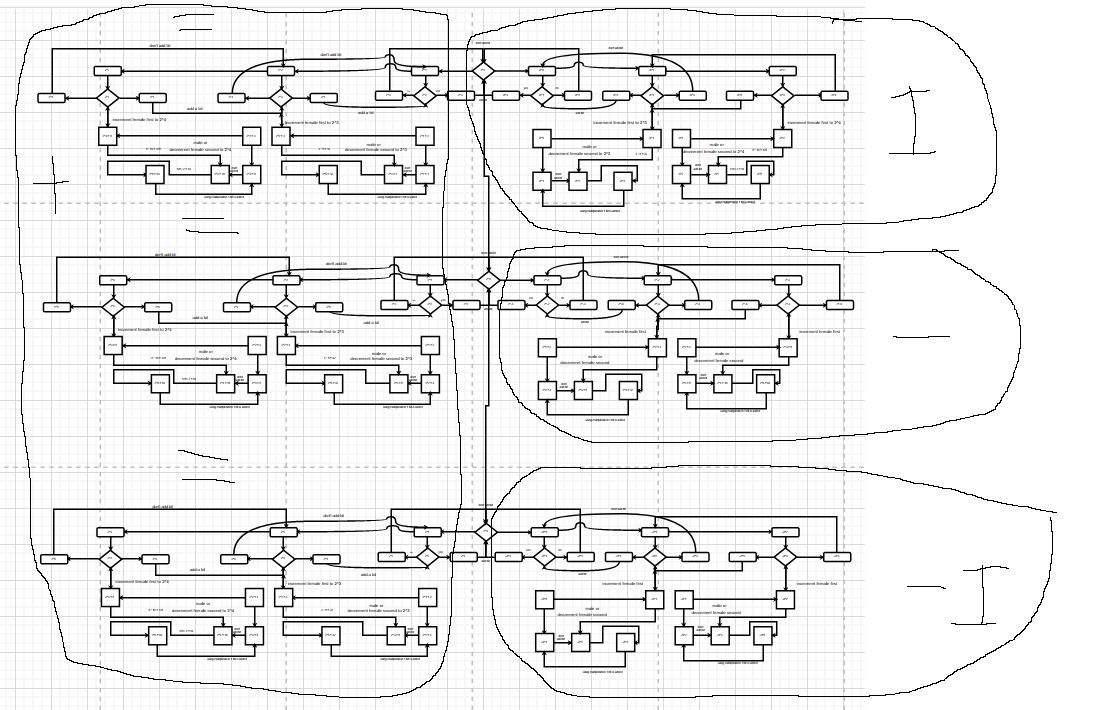• i wrote out the formula for the fighting methods ( https://forums.oculusvr.com/community/discussion/comment/721602/#Comment_721602 ) below using my previous posts math, no diagram though since that's extra work and I'm not prepared to do that right now;

starts at 2:
[
i = forward
- [2i^0 = 2] = 2^1 = left
- [2i^1 = 2i] = forward
- [2i^2  = -2] = -2^1 = right
- [2i^3 = -2i] = reverse
- [2i^4 = 2] = 2^1 = left
- [2i^5= 2i] = forward

-i = reverse
- [-2i^0 = -2]  = -2^1 = right
- [-2i^1 = - 2 i] = reverse
- [-2i^2 = 2] = 2^1 = left
- [-2i^3 = 2i] = forward
- [-2i^4 = -2] = -2^1 = right
- [-2i^5 =  -2i] = reverse
]

starts at 0:
[
positive = left
- [2i^0 = 2] = 2^1 = left
- [2i^1 = 2i] = forward
- [2i^2  = -2] = -2^1 = right
- [2i^3 = -2i] = reverse
- [2i^4 = 2] = 2^1 = left
- [2i^5= 2i] = forward
- [2i^6 = -2] = -2^1 = right

negative = right
- [-2i^0 = -2]  = -2^1 = right
- [-2i^1 = - 2 i] = reverse
- [-2i^2 = 2] = 2^1 = left
- [-2i^3 = 2i] = forward
- [-2i^4 = -2] = -2^1 = right
- [-2i^5 =  -2i] = reverse
- [-2i^6 = 2] = 2^1 = left
]

originally i starts at 2 = memory, 0 is memory for i.

as i goes right, i has to go into the negative real number.
and as -i goes left, -i has to go into the positive real number.

there is no i there is real numbers, (0/no) imaginary memory here.

memory to no memory.

there is the advantage of having memory: how does i start.

with guns you have:
- [-i < i] = strafing left > right (left or right hand orientates which side is positive and which is negative which may switch left < right)
- [-i < i] = moving forward >  [strafing left > strafing right (see above comment)] > backing up

i [i, -i], -i

with swords:
[i = person 1 attacks][i, -i = person 2 easy defence], [-i = person 2 hard defence]
person 1 switches i into the real numbers, having no memory.
person 2 still had i at the real number, which can attack person 1 now that person 2 i is now in the imaginary and so has memory.
all person 2 has to do is to move i into the imaginary which is one move, which person 1 has to move i through both real then imaginary.

as person 2 does this change into the imaginary there is the i > -i to consider on which method is easiest to do.
go forward is easiest you do this, then stay in the real and counter the person 1 you consider the other person, or move backward which is the harder imaginary as it involves other people or things outside your power you consider the environment.

and that is sword fighting.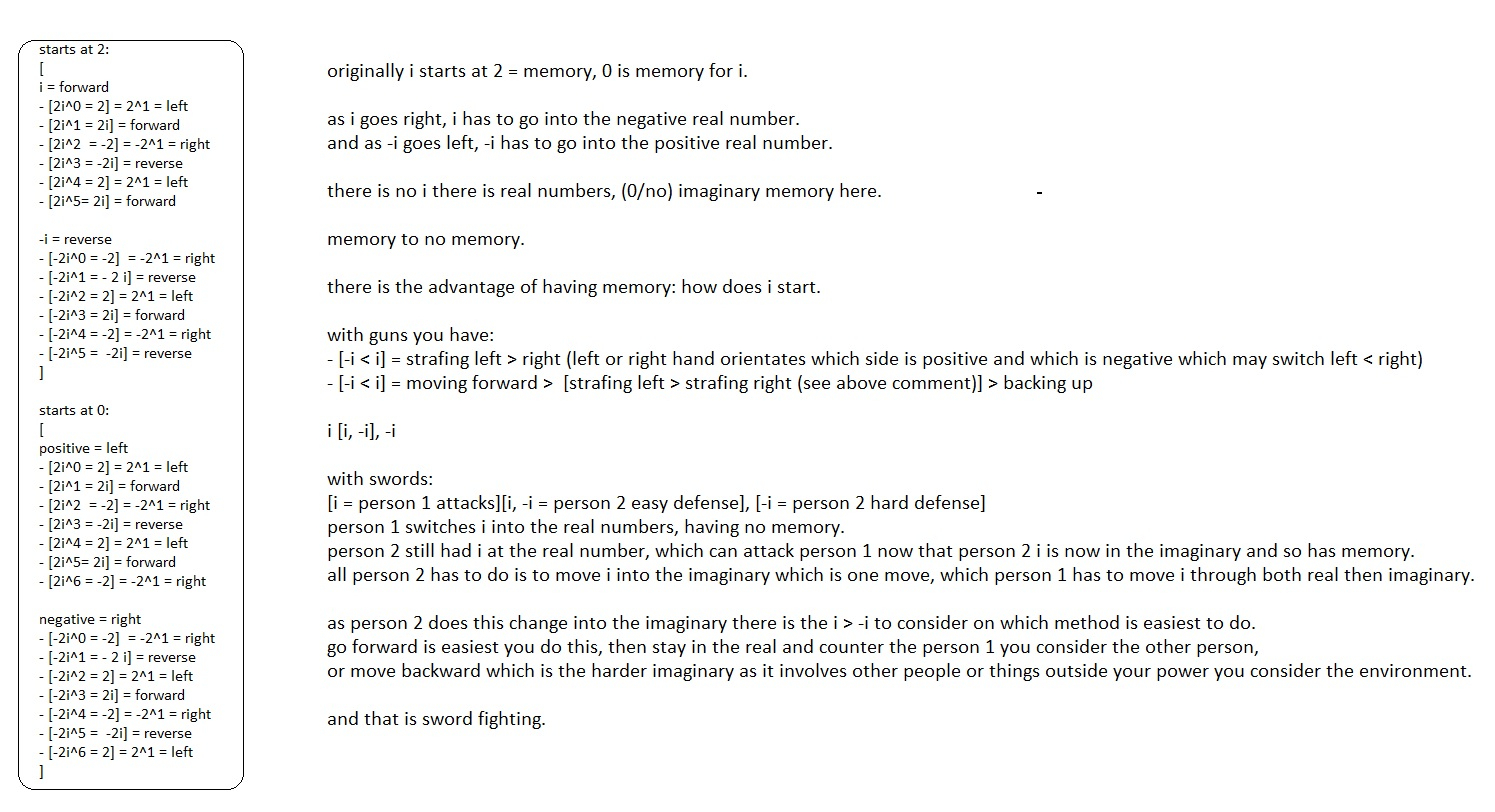i imagine drawing this out on the huge diagram i posted in my previous post would be horrible work to do, so i probably won't do it, so there you go, AI for fighting.

now i have the theory diagrammed it can be coded up as pseudo code, if i do the final diagram that is. and I'm no a good programmer and don't know how to code AI or neural networks and i gave up game programming because i got lazy. there's only so much i can be expected to do...

• edited January 18
person 1

starts at 2:
[
i = forward
- [2i^0 = 2] = 2^1 = left
- [2i^1 = 2i] = forward
- [2i^2  = -2] = -2^1 = right
- [2i^3 = -2i] = reverse
- [2i^4 = 2] = 2^1 = left
- [2i^5= 2i] = forward

person 2

starts at 0:
[
positive = left
- [2i^0 = 2] = 2^1 = left
- [2i^1 = 2i] = forward
- [2i^2  = -2] = -2^1 = right
- [2i^3 = -2i] = reverse
- [2i^4 = 2] = 2^1 = left
- [2i^5= 2i] = forward
- [2i^6 = -2] = -2^1 = right

person 1

continues at 0:
[
positive = left
- [2i^0 = 2] = 2^1 = left
- [2i^1 = 2i] = forward
- [2i^2  = -2] = -2^1 = right
- [2i^3 = -2i] = reverse
- [2i^4 = 2] = 2^1 = left
- [2i^5= 2i] = forward
- [2i^6 = -2] = -2^1 = right
]

person 2

continues at 2:
[
i = forward
- [2i^0 = 2] = 2^1 = left
- [2i^1 = 2i] = forward
- [2i^2  = -2] = -2^1 = right
- [2i^3 = -2i] = reverse
- [2i^4 = 2] = 2^1 = left
- [2i^5= 2i] = forward

continues at 0:
[
positive = left
- [2i^0 = 2] = 2^1 = left
- [2i^1 = 2i] = forward
- [2i^2  = -2] = -2^1 = right
- [2i^3 = -2i] = reverse
- [2i^4 = 2] = 2^1 = left
- [2i^5= 2i] = forward
- [2i^6 = -2] = -2^1 = right

negative = right
- [-2i^0 = -2]  = -2^1 = right
- [-2i^1 = - 2 i] = reverse
- [-2i^2 = 2] = 2^1 = left
- [-2i^3 = 2i] = forward
- [-2i^4 = -2] = -2^1 = right
- [-2i^5 =  -2i] = reverse
- [-2i^6 = 2] = 2^1 = left
]

continues at -2:

-i = reverse
- [-2i^0 = -2]  = -2^1 = right
- [-2i^1 = - 2 i] = reverse
- [-2i^2 = 2] = 2^1 = left
- [-2i^3 = 2i] = forward
- [-2i^4 = -2] = -2^1 = right
- [-2i^5 =  -2i] = reverse
]

this would be the algorithm for sword fighting, person 1 starts at attacking using the gun fighting logic. person 2 counters using sword fighting logic.

this is an algorithm. you see the final four groups are a example of this logic; i [i, -i], -i, you need to move, you need to counter, you need to give and receive third party support. if balanced properly each member supports the other and denies this support to their enemy and so wins as a group.

its a scale balance. i can't express my thoughts on this but the math is true. maybe later if i can i will verbalize it better...

i didn't draw this on the diagram i don't know how to.

• edited January 29
I got around to adding in some math to the initial chart i made. here's the math, the diagram is the same as before when i first started drawing diagrams but it includes math;

(total number of people) (the proportion of all possibilities where h is true) (the proportion of those events where e is also true)
-----------------------------------------------------------------------------------------------------------------------------------------
(total number of people) (the proportion of all possibilities where h is true) (the proportion of those events where e is also true) +
(total number of people) (the proportion of all possibilities where h is not true) (the proportion of those events where e is also true)

(total number of people)(p(h))(p(e | h))
--------------------------------------------------------------------------------------------
(total number of people)(p(h))(p(e | h)) + (total number of people)(p(not h))(p(e | not h))

= posterior = (p (h|e)) = belief about the hypothesis after seeing the evidence

first calculation
(total number of people)(p(a))(p(b | a)) = [(p(evidence))(p(hypothesis | evidence))]
-------------------------------------------------------------------------------------------
(total number of people)(p(a))(p(b | a)) + (total number of people)(p(not a))(p(b | not a))
= = p(a|b) = p(evidence | hypothesis)
= a > b = evidence > hypothesis

second calculation
(total number of people)(p(b))(p(a | b)) = [(p(hypothesis))(p(evidence | hypothesis))]
-------------------------------------------------------------------------------------------
(total number of people)(p(b))(p(a | b)) + (total number of people)(p(not b))(p(a | not b))
= p(b|a) = p(hypothesis | evidence)
= b < a = hypothesis < evidence

2 ^ 0 = 1 = 1 x 1 area

hypothesis = 2 ^ 2 = 4 = (the proportion of all possibilities where h is not true), from first calculation

evidence = 2 ^ 4 = 16 = (the proportion of all possibilities where h is not true), from second calculation

hypothesis = 2 ^ 3 = 8 = (the proportion of all possibilities where h is true), from second calculation

evidence = 2 ^ 1 = 2 = (the proportion of all possibilities where h is true), from first calculation

hypothesis = 2 ^ -2 = 0.25 = (the proportion of all possibilities where h is not true), from first calculation

evidence = 2 ^ -4 = 0.0625 = (the proportion of all possibilities where h is not true), from second calculation

hypothesis = 2 ^ -3 = 0.125 = (the proportion of all possibilities where h is true), from second calculation

evidence = 2 ^ -1 = 0.5 = (the proportion of all possibilities where h is true), from first calculation

i probable made a math typo when i labeled 2^-1 as -2, i didn't bother to think it through i was too lazy. sorry. i double checked this time though and fixed the typo. my fault.

i made get around to labeling the entire graph if I'm not too lazy to do so at some time later on, but for now here's the Bayes theorem added to my diagram.

• audacity, add track, select all, effect, change pitch.

sound 1
pitch tab
from c 1 to c sharp d flat
semitone half steps 1

frequency tab
from 32.703 to 34.648 Hz
percent change 5.946

a different value for the pitch tab and frequency tab now;

sound 2
pitch tab
from c 1 to d
semitone half steps 2

frequency tab
from 32.703 to 36.708 Hz
percent change 12.246

step 1, sum of two sounds
second sound is sound 1
step 2, sum of two sounds
second sound is sound 2

step 1 and 2 precede logic.

logic 1 = good
sum of two sounds
second sound is sound 1 = 2 ^ 2

sum of two sounds
second sound is sound 2 = 2 ^ 3

pattern that 2^2 and 2^3 fit into:

hypothesis = 2 ^ 2 = 4 = (the proportion of all possibilities where h is not true), from first calculation

evidence = 2 ^ 4 = 16 = (the proportion of all possibilities where h is not true), from second calculation

hypothesis = 2 ^ 3 = 8 = (the proportion of all possibilities where h is true), from second calculation

evidence = 2 ^ 1 = 2 = (the proportion of all possibilities where h is true), from first calculation

this is how sound conveys first a mimicry, then a logic that holds different values of sound to show the logic in relation to mimicry.

without mimicry the logic has no value, so to read a sound value from a person you then find their step 1, 2, sounds then place that in the Bayes theorem map i wrote then you see how the sound is logical and how to relate to that sound by outputting a logical mimicry sound.

and different people know different mimicry sound values which gives them personality other people identify them by.

and that's how to mold a chat bot using natural language. that's how people think.

• Posts: 2,211Valuable Player
edited February 11
PCVR: CV1 || 4 sensors || TPcast wireless adapter || MamutVR Gun stock V3
PSVR: PS4 Pro || Move Controllers || Aim controller
WMR: HP Reverb
• Posts: 15,006Valuable Player
Are you a fan of the Myst games? Check out my Mod at http://www.mystrock.com/
• edited February 22
i wrote up some pseudo code for a better chat bot logic, its not perfect but this is how to integrate my diagram with the chat bot to generate logical conversation;

(angle + [360 * n])

person says = "do you know what 2 + 2 is?"
(angle + [360 * n]) = (do you know what + angle) + (360 * [(2 + 2) = positive coterminal angle])
machine replies = "yes"

person says = "do you know andy?"
(angle + [360 * n]) = (do you know + angle) + (360 * [(andy) = negative coterminal angle])
machine replies = "no."

person says = "i am andy."
(angle + [360 * n]) = (i am + angle) + (360 * [(andy) = positive coterminal angle])
machine replies = "yes"

person says = "who is andy?"
machine processes =
(angle + [360 * n]) = (who is + angle) + (360 * [(andy) = positive coterminal angle])
(angle + [360 * n]) = (i am + angle) + (360 * [(andy) = positive coterminal angle])
(angle + [360 * n]) = (do you know + angle) + (360 * [(andy) = negative coterminal angle])
(angle + [360 * n]) = (do you know (-) + i am (+) + who is (+) + angle) + (360 * [(andy) = (-, +, +) = positive coterminal angle])

///////////

what the above example of a chat bot does is two things;
- with no operator (+,-,*,/, ^,())
- first no repeated words = 2^3, second there's repeated words = 2^2

- once you have a repeated word, this repeated word acts like a operator, with a operator you have a positive coterminal angle result.

that shows the positive integer values. now assume you can mix and match the formula to stretch into the other three sections of the diagram (negative integers, positive imaginary, negative imaginary).

assuming you can do so by mixing and matching the positive integer formula, here is my best guess on how to do so.

positive integer values;
(angle + [360 * n]) = (do you know (-) + i am (+) + who is (+) + angle) + (360 * [(andy) = (-, +, +) = positive coterminal angle])

negative integer values;
(angle + [360 * n]) = (do you know (+) + i am (-) + who is (-) + angle) + (360 * [(andy) = (+, -, -) = negative coterminal angle])

positive imaginary values;
(angle + [360 * n]) = (
([do you know (-) +] = null)
i am (+) + who is (+) + angle) +
(360 * [(andy) = (- = null, +, +) = positive coterminal angle])

negative imaginary values;
(angle + [360 * n]) = (
do you know (-) +
([i am (+) + who is (+) +] = null)
angle) +
(360 * [(andy) = (-, + = null, + = null) = negative coterminal angle])
• i updated my previous reply.
• I’m not sure what I just read but I am impressed 😀

Hahah same here.
• edited March 21
i redid the math so it fits the basic template and makes sense on a grade school logic so it makes sense.

I merged in the law of sines. next I will try to merge in the law of cosines but that being more complicated will take much thinking. but I think I can do it, in the mean while here is the new diagram with the new law of sines values.

correction I made a typo by not writing in the - values of binary. in the sines diagram, here is the good version along with the law of cosines;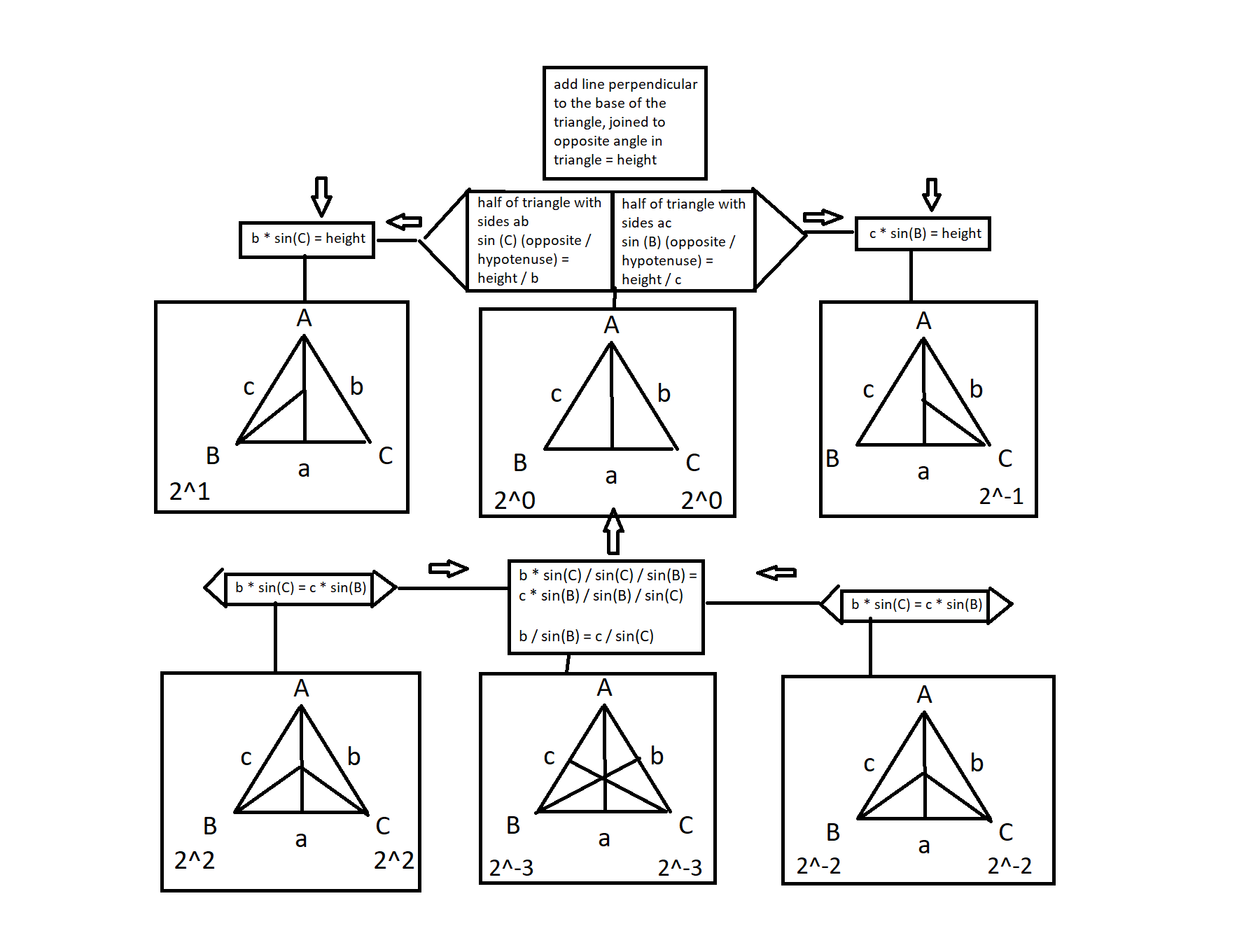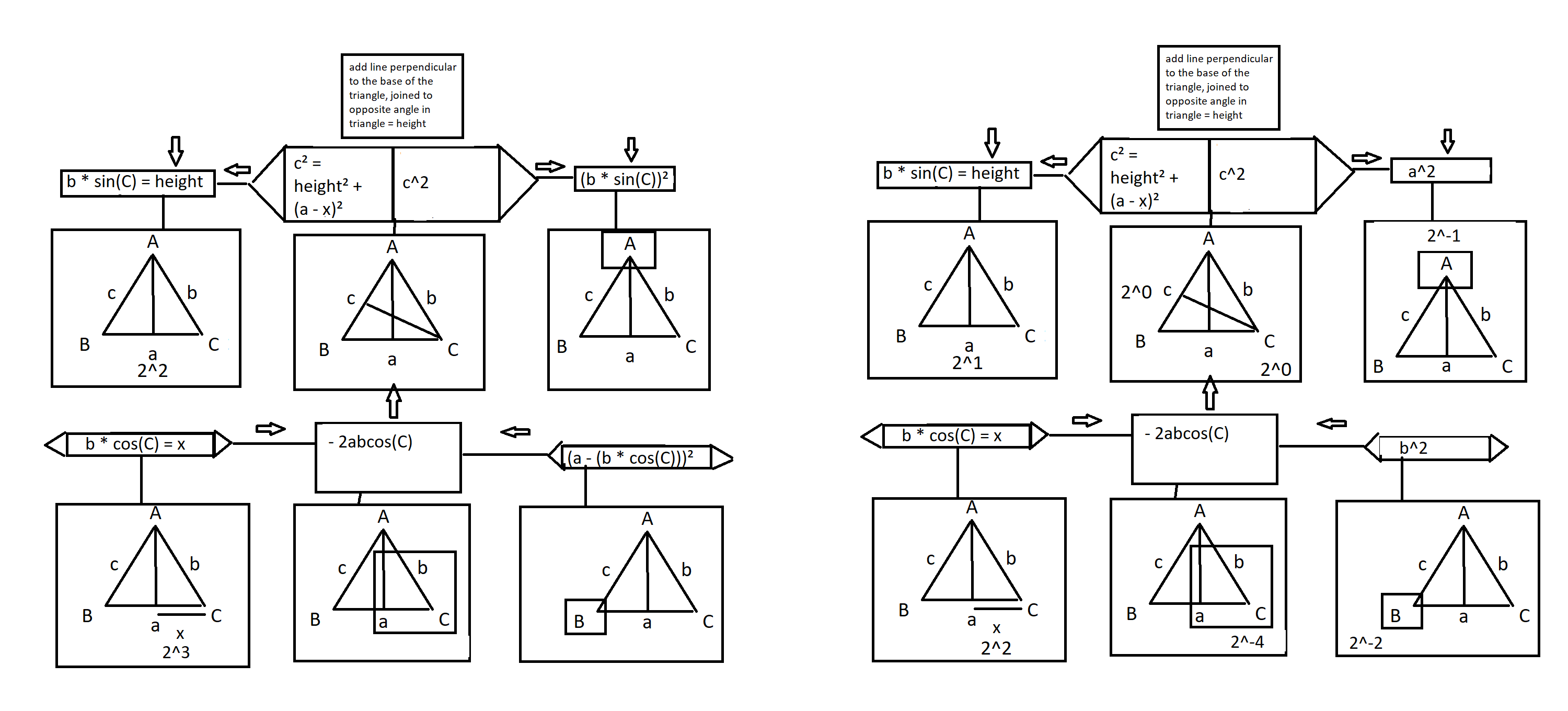as the imaginary positive and negative values only use one group, like the law of sines, but we see the law of cosines use of two groups mixing both positive and negative values. see the sword technique for an example.

here we see the height going to the angle A to the side A, but say you need to find a different side than side c, then you use a different side to put the height on, and that's where you get the use of the cosine using the positive and negative imaginary values.

• Posts: 2,899Valuable Player
nobody cares. so it doesnt matter. but its for bot decisions which is sort of for ai and games, i guess. but it doesnt matter, nobody cares. no worries friendo.

I have no idea what all that math means, but if it makes the bots in Onward a lot better than they are, Send that to Downpour so they can improve the bots.
• Interesting theory
• edited March 23
falken76 said:
nobody cares. so it doesnt matter. but its for bot decisions which is sort of for ai and games, i guess. but it doesnt matter, nobody cares. no worries friendo.

I have no idea what all that math means, but if it makes the bots in Onward a lot better than they are, Send that to Downpour so they can improve the bots.
i play call of duty modern warfare and see an algorithm in the way the opponent manages his time in the game.

its similar to the Galapagos island lizard inhabitants. the lizards i speak of live on the Galapagos islands and they eat the seaweed a fair distance in the water. they have to leave their cliffs and swim out then dive deep to get the seaweed.

its a marathon effort every time they have to eat.

back in the call of duty game, in a team of 6  vs 6 the opponent getting 1 life per round, treks out a fair distance at the start of the round. a marathon distance, every round, then they pop out of their secret path and they meet me. i travel only a stones throw away from my spawn point, crouch down, and wait like a fisherman. 9 times out of ten the opponent does this.

now what does this have to do with my trigonometry math you ask? the team alpha = sine, the team bravo = cosine.

team bravo goes on a trek out and they meet team alpha. where, inside of cosine at 2^0.

assuming you have multiple paths team bravo can travel, you have each path = some 2^ value increment the power for each additional path.

that means all paths but 1 = 0, the path that = 1 is the path team alpha should meet team bravo. where does team alpha meet team bravo when 2^ = 1? when team alpha = 1. team alpha can meet team bravo so that team alpha is 0 then there is no ability to correctly take advantage of team bravo even though team alpha headed off team bravo at 2^0 in the cosine diagram.

and that is how the math fits into the game and the lizard story.

looks like this;

team bravo:
2^0 = 0
2^1 = 0
2^3 = 1
2^4= 0

(team bravo == 1 and team alpha == 1)
{
team alpha = 1
}

then assume you have multiple points team alpha has to = 1, moving past the first alpha = 1, then bravo is set to 1 at some point where the cosine = 2^0, there alpha has to equal 1 too.

this repeats until alpha doesn't have to move to a new alpha.

• i thought about using this method to start doodling to make a amateur chat bot and how would i do that.

i went back to an idea i had about clearing space while playing the call of duty modern warfare game. in that game to clear space you have a 1 0 algorithm where 0 = flat and 1 = not flat or a spot someone can be in.

as 0 approaches 1, 0 has two methods, 0 is alpha in my previous example, 1 is beta. beta might be in 1 it might not be, so alpha or 0 has to clear the area using the flat method, this is you don't allot value or yourself to the spot you peek, how you peek is you slink around. this mathematically means (1 =1) , and (0 = 0), 0 does not = 1. the math functions like a gun trigger once pulled it fires, but this is symmetrical what works for 0 works for 1 and then they both have use for the trigger to deal with the other number.

so 0 using flat goes to clear 1, and 1 having a trigger can let 0 meet the trigger before clearing 1. if 0 meets the trap that is the trigger then 0 = 1 and this is false for the flat alpha and alpha gets tagged/loses.

so alpha has to be sure that 0 clearing 1 has no trigger it can fall into and that means when alpha or the sine fixes the cosine, then the solution doesn't create more problems than it fixes.

now how would that be useful for chat bots i thought and here is the example dialog i thought up. its not a fancy idea but it uses the basic logic i detailed.

person to chat bot: hello = 1
chat bot to person: hello = 0
person to chat bot: an "orange" is "orange"  = 1 // written in code as (orange = variable) = (value = orange)
chat bot to person: i don't know what an orange is = 1 imaginary number cosine // written in code as (orange = variable)
person is chat bot: an orange is a:) a fruit, b:) a color // written as something grouping the word orange and the word orange, maybe a list i don't know...from here i get lost coding it
chat bot to person: an orange (fruit) = 1, is orange (color) = trigger = 1 cosine real number
person to chat bot: an orange is orange = 1
chat bot to person: orange (trigger) = 0
person to chat bot: hello orange: = 1
chat bot to person: hello orange: 0 (fruit = 1), orange is orange: 0 (orange = color)

so that's my template pseudo code to write my chat bot bot when it gets complicated i get lost in thinking of how to code it so i'll leave it here for food for thought.

Joffrey has 0 = 1 and had to be taken care of;

• Posts: 15,006Valuable Player
edited March 28
person to chat bot: an "orange" is "orange"  = 1 // written in code as (orange = variable) = (value = orange)

Every object needs a type.

• Orange.typeof(color)
Orange.TypeOf(fruit)

chat bot to person: hello orange: 0 (fruit = 1), orange is orange: 0 (orange = color)
Joffrey has 0 = 1 and had to be taken care of
Joffrey needs to write this 100 times on the Whiteboard:
An object is an abstract data type with the addition of polymorphism and inheritance.

Are you a fan of the Myst games? Check out my Mod at http://www.mystrock.com/
• i rethought the math and came up with an abstract take on it, since i forgot what i meant the first time and i didn't write the idea out clearly the first time.

type a = (0 = 0) = alpha
type b = (1 = 1) = beta
type c = (1 != 0) =  alpha = type a, and beta = type b

type a = type c != type b = type c, because type c returns type a to type a, and type b to type b.

the trigger algorithm then goes like this;

type a = 1, type b = 1

// trigger for type b
if type b = type c:
{type a != type c
type c != type a
type c = type b}

if there being a (type c that = type b) that type c is outside of the awareness of type a:
{type a != type b
trigger for type b
trigger for type a}

that is the math for the war game call of duty modern warfare, now how to write this into my chatbot  idea is the next step, i shall try to do this now, below;

person to chat bot: hello = type beta
chat bot to person: hello = type alpha
person to chat bot: an "orange" is "orange"  = 1 // written in code as (orange = variable) = (value = orange)
Orange.TypeOf(fruit) = Orange.typeof(color)

chat bot to person: i don't know what an orange is = 1 imaginary number cosine // written in code as (orange = variable)
person is chat bot: an orange is
{Orange.TypeOf(fruit)
Orange.typeof(color)}

chat bot to person: an orange (fruit) = 1, is orange (color) = trigger
{side a = angle A, law of cosine find side a = Orange.TypeOf(fruit)
side b = angle B, law of cosine find side b = Orange.TypeOf(color)}

person to chat bot: an orange is orange = 1
chat bot to person: orange (trigger) = 0
{side a = angle A, law of cosine find side a = Orange.TypeOf(fruit)
side b = angle B, law of cosine find side b = Orange.TypeOf(color = trigger)}
Orange.TypeOf(fruit) = Orange.typeof(color) // going from finding side b then side a

person to chat bot: hello orange: = 1
chat bot to person: hello orange: 0 (fruit = 1), orange is orange: 0 (orange = color)
Orange.TypeOf(fruit) = Orange.typeof(color): // going from finding side b then side a
{side a = angle A, law of cosine find side a = Orange.TypeOf(fruit)
side b = angle B, law of cosine find side b = Orange.TypeOf(color)} =
{side b = angle B, law of cosine find side b = Orange.TypeOf(color)
side a = angle A, law of cosine find side a = Orange.TypeOf(fruit)} // i treat the left side of the equal sign  like its 1 variable, not sure how to write that into code but this is the best i can do as a type of pseudo code

logical conclusion:
{side a = angle A, law of cosine find side a = Orange.TypeOf(fruit)
side b = angle B, law of cosine find side b = Orange.TypeOf(color = trigger)}
!=
side c = angle C, law of cosine find side c = apple.TypeOf(fruit)

thats my try at the chat bot.